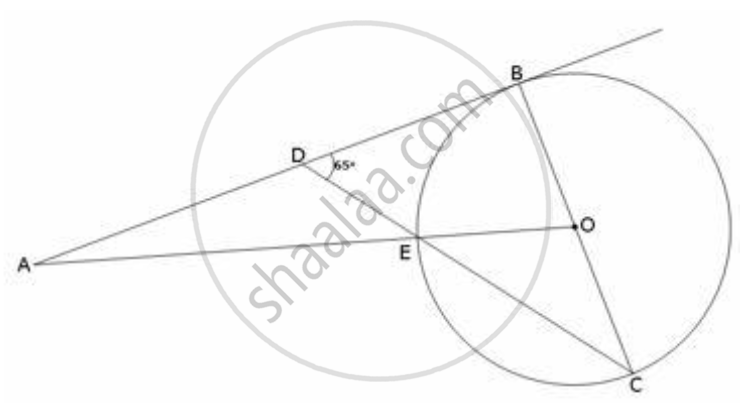Share

# In the Following Figure, O is the Centre of the Circle and Ab is a Tangent to It at Point B. ∠Bdc = 65°. Find ∠Bao. - Mathematics

Course

#### Question

In the following figure, O is the centre of the circle and AB is a tangent to it at point B. ∠BDC = 65°. Find ∠BAO.

#### SolutionForm the given figure OB is the radius and therefore in triangle BDC

∠DBC + ∠BDC + ∠BCD = 180^@

=> 90^@ + 65^@ +  ∠BCD = 180^@

=> ∠BCD = 25

Now, OE = OC = radius,  ∠OEC = ∠OCE = 25° (as ∠OCE = ∠BCD)

=> ∠AED = ∠OEC = 25°   (Vertically opposite angles)

Also, ∠ADE = 180^@ - 65^@ = 115^@

Therefore in triangle AED

∠BAO = 180^@ - 115^@ - 25^@ = 40^@

Is there an error in this question or solution?